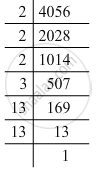Advertisement Remove all ads

# By What Number Should Each of the Following Numbers Be Multiplied to Get a Perfect Square? Also, Find the Number Whose Square is the New Number. 4056 - Mathematics

By what number should each of the following numbers be multiplied to get a perfect square? Also, find the number whose square is the new number.

4056

Advertisement Remove all ads

#### Solution

4056 = 2 x 2 x 2 x 3 x 13 x 13Grouping them into pairs of equal factors:
4056 = (2 x 2) x (13 x 13) x 2 x 3
The factors at the end, 2 and 3 are not paired. For a number to be a perfect square, each prime factor has to be paired. Hence, 4056 must be multiplied by 6 (2 x 3) for it to be a perfect square.
The new number would be (2 x 2) x (2 x 2) x (3 x 3) x (13 x 13).
Furthermore, we have:
(2 x 2) x (2 x 2) x (3 x 3) x (13 x 13) = (2 x 2 x 3 x 13) x (2 x 2 x 3 x 13)
Hence, the number whose square is the new number is:
2 x 2 x 3 x 13 = 156

Is there an error in this question or solution?
Advertisement Remove all ads

#### APPEARS IN

RD Sharma Class 8 Maths
Chapter 3 Squares and Square Roots
Exercise 3.1 | Q 7.5 | Page 4
Advertisement Remove all ads

#### Video TutorialsVIEW ALL 

Advertisement Remove all ads
Share
Notifications

View all notifications

Forgot password?
Course